Disclaimer: This is an example of a student written essay.

Any opinions, findings, conclusions or recommendations expressed in this material are those of the authors and do not necessarily reflect the views of UKEssays.com.

# Algorithm for Robot Navigation Without Collisions

 ✅ Paper Type: Free Essay ✅ Subject: Computer Science ✅ Wordcount: 1742 words ✅ Published: 19th Apr 2018

ALGORITHM FOR ROBOT NAVIGATION AT ENVIRONMENT WITHOUT COLLISION

ALGORITHM REPRESENTATION FOR NAVIGATION OF MOBILE ROBOT WITHOUT OBSTACLE COLLISON

Mobile robot It is a kind of robot that has the ability to travel Relative to the environment (i.e. locomotion), and one of the actuators of the robot is the locomotive system

This chapter of my bachelor thesis is to develop algorithms that will help the autonomous mobile robot in visual navigation. g the robot. Then, the robot tries to understand their environment to extract data from a sequence of image data, in this case, optical, and then uses this information as a guide for the movement. The strategy adopted to avoid collisions with obstacles during movement – a balance between the right and left optical flow vectors.

If you need assistance with writing your essay, our professional essay writing service is here to help!

An integral part of any navigation scheme is the desire to reach a destination and do not get lost or bump into any of the objects. There may be other restrictions on a given route, such as speed limits or zones of uncertainty, where in theory, of course, can pave the path, but not desirable. Often, the way is to move the robot autonomously planned, ie on the basis of previous input and without interference in real time. It can work effectively, but only on condition that the environment is perfectly known and does not change and the robot can travel on the route perfectly. However, in the real world everything is much more complicated.

• Location of robot
• Environment perception and his model
• Methods of traffic planning
• Robot motion control algorithms

The problem of mobile robot navigation is a very complex issue characteristic at both ends. The implementation of tasks by moving a mobile robot requires obtaining information about the surrounding-limiting environment – hence the importance of having AD sensory system that allows the observation of the environment and its perception, For this purpose, both simple rangefinder systems and contact sensors, which correspond with collision detection.

Using a constant speed of 4m/s for the algorithm and a step size of 0.125m which was obtained by the multiplication of the speed by interval in which information is received.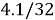= 0.125m. The algorithm is given below.

1. Set iteration values K equals K =1. Tolerance factor δ
2. Using the following sequence inside the loop for K
• Evaluating Hessian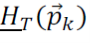and the gradient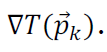and also checking for positive definite of hessian matric
• Determine Newton Direction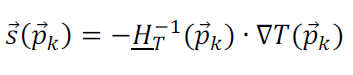• Normalize Newton Direction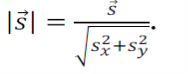• Determine step size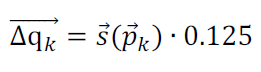• Determine new point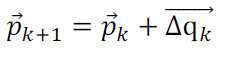3. If ||ΔT||<δ, if not set K=1 And repeat step 2.otherwise terminate

But considering the above algorithm it’s still going to encounter some problems. For example saw tooth pattern that occurs at the along the path, shown below: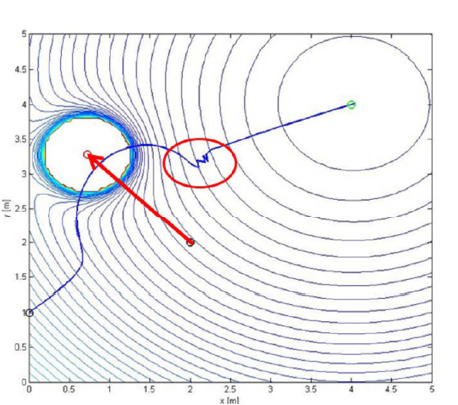Saw-tooth pattern

Saw-tooth happens due to fixed step size at some point in the navigation of the mobile robot reduction in step size is necessary which also means reduction in the speed of the robot . The reason for this effect is because the present point of the robot is not always the best point possible. Meaning that point after that will guide the path back, resulting in a saw=tooth pattern zig -zagging along the path. The reason this problem occurs is because the robot has a constant speed.

To determine the new point of the robot the speed and acceleration needs to be known if we have a speed of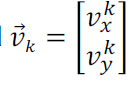and an acceleration of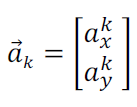The constraints are |speed|<= 4m/s and |acceleration|<=5m/s. the time parameter T of the robot specification gives the constraints. Now the speed of the robot can be determined by each step at each time stop K+1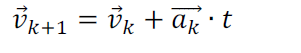Now starting speed will be set has speed(K=0)=0m/s, which means is assumed that robot is in a static state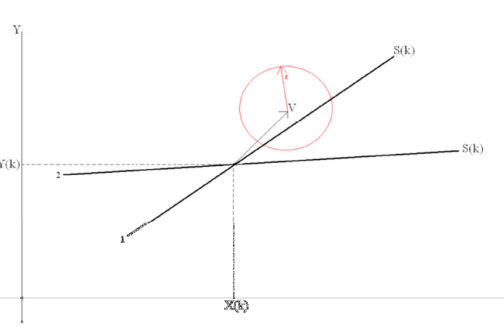Determining position of robot

All points in the line represent the Newton’s Direction. Robot needs to move to one of its point so we can determine the speed and acceleration of robot

OVERSHOOT SCENARIO:

This is a scenario when the acceleration that is generated is not large enough to get to the point on the newton direction, solution to this can’t be found, the only way out is that the point closer to the line will move .I.e. line perpendicular to the newton’s direction must be found and the lie should intercept in the center.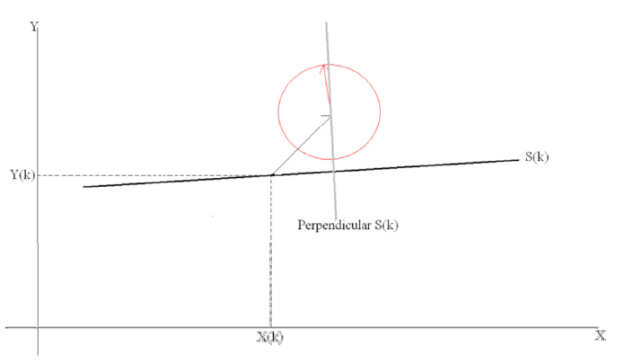Now considering the new algorithm

1. Setting values at start point, target point and obstacle location

MATHEMATICAL BACKGROUND OF ALGORITHM

FUNCTION OF TARGET:

Every robot has its starting point and it has its destination that to say its target point and to accomplish this task it needs a target function: Target function is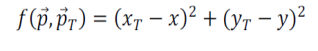Where the position of the mobile robot is at present is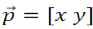and the destination of mobile robot is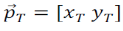. A mobile robot has reached its minimum function when current position of the robot is equal to the target position.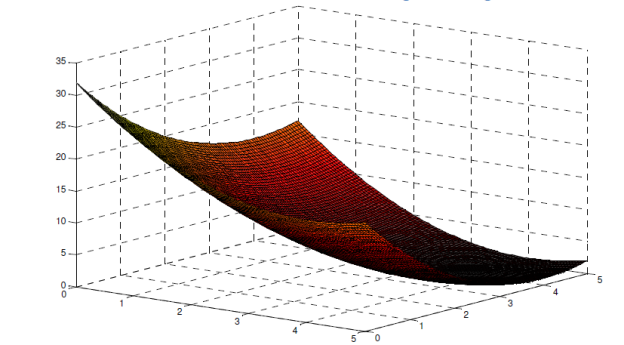Fig 1: Position of Target

BOUNDARY FUNCTION:

Every Mobile robot has its environment and areas that are out of mobile robots environment is therefore represented with a boundary. What the boundary represents is the size, shape and location of an object. Boundary function and function of target will both give an optimization problem when finding the minimum.

BARRIER FUNCTION:

The most difficult part of mobile robot navigation is generating its path without going out of its environment that is where the barrier function comes in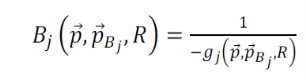The barrier function and the target function are added up, and this leads to the following function: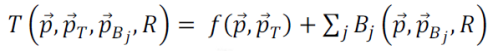PENALTY FUNCTION:

What the penalty function does is that it controls the importance of obstacles on the path of a mobile robot. It show if an obstacle is of high priority or isn’t. This is where distance comes to play how close the obstacle to the robot is to the obstacle. When calculating the penalty function of a mobile robot the most important obstacles are the obstacles closer to the robot. The penalty function is obtained by the calculation of the distance between the obstacle and the mobile robot. The result of the calculation shows the increases or decreases considering the movement of the robot away or towards the obstacle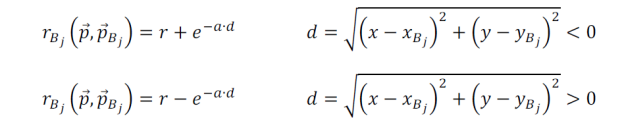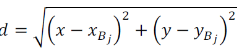This represents the variation is the distance between the obstacle and mobile robot.

NEWTON DIRECTION:

Mobile robot optimization is very important in robot navigation. Choosing the most efficient path to follow to from robot’s current position to the target point around its environment, this is called Newton method. Newton direction is calculated by the optimal direction in which a step should be taken, ithis is given in the equation below: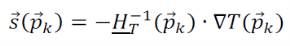Where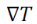is the gradient of target function and the inverse of hessian matrix is: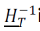which is used to describe the second order derivative of the function of target, that is evaluated at point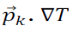(delta t) is used in describing the change in the first order derivative of function of target.

THEORITICAL EXPERIMENT

After considering the algorithm it will be right to do some experiments based on the algorithm to investigate and test whether it does what we want it to. I will be using static obstacles to test.

ONE STATIONARY OBSTACLE:

Stationary point;

View all

## DMCA / Removal Request

If you are the original writer of this essay and no longer wish to have your work published on UKEssays.com then please:

Related Services

Prices from

OMR57

Approximate costs for:

• 1000 words
• 7 day deliveryHumanity University

Dedicated to your worth and value as a human being!

Related Lectures